Welcome to our website!

# Dynamic Arrays in Excel

Dynamic Dynamic Arrays Excel Range Names

Overview

Dynamic Arrays in Microsoft Excel is a game changer. To make it simple Dynamic Arrays (“DA”) allow you to work with multiple values at the same time.

This major change is expected to change the design and construction of excel models.

Dynamic Arrays are only available in Microsoft Excel 365. The functions that are available are presented below:

• FILTER
• RANDARRAY
• SEQUENCE
• SORT
• SORTBY
• UNIQUE

You can check the free file with the worked examples here.

Let us get our hands dirty with some examples. We start with the following data as per below (the data has 12 500 rows, and 7 columns).

Table 1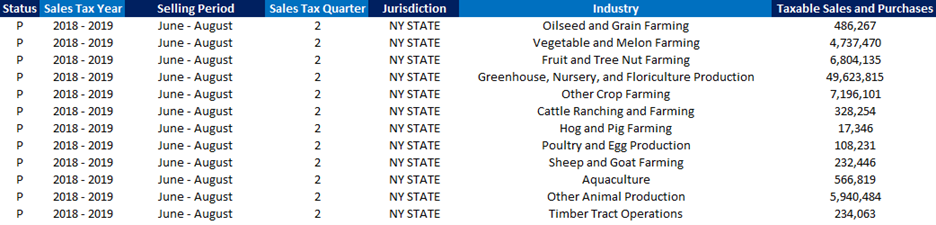UNIQUE

Below we are using the new UNIQUE function to extract the unique values from this data set.

This will give you the unique values for Status, Sales Tax Year, Selling Period, Sales Tax Quarter, Jurisdiction, and Industry.

If any value in the data set is changed the UNIQUE formula will automatically update the results.

To produce the results below, we are using a single formula for each column (B9, C9, D9, E9, F9, G9)

Table 2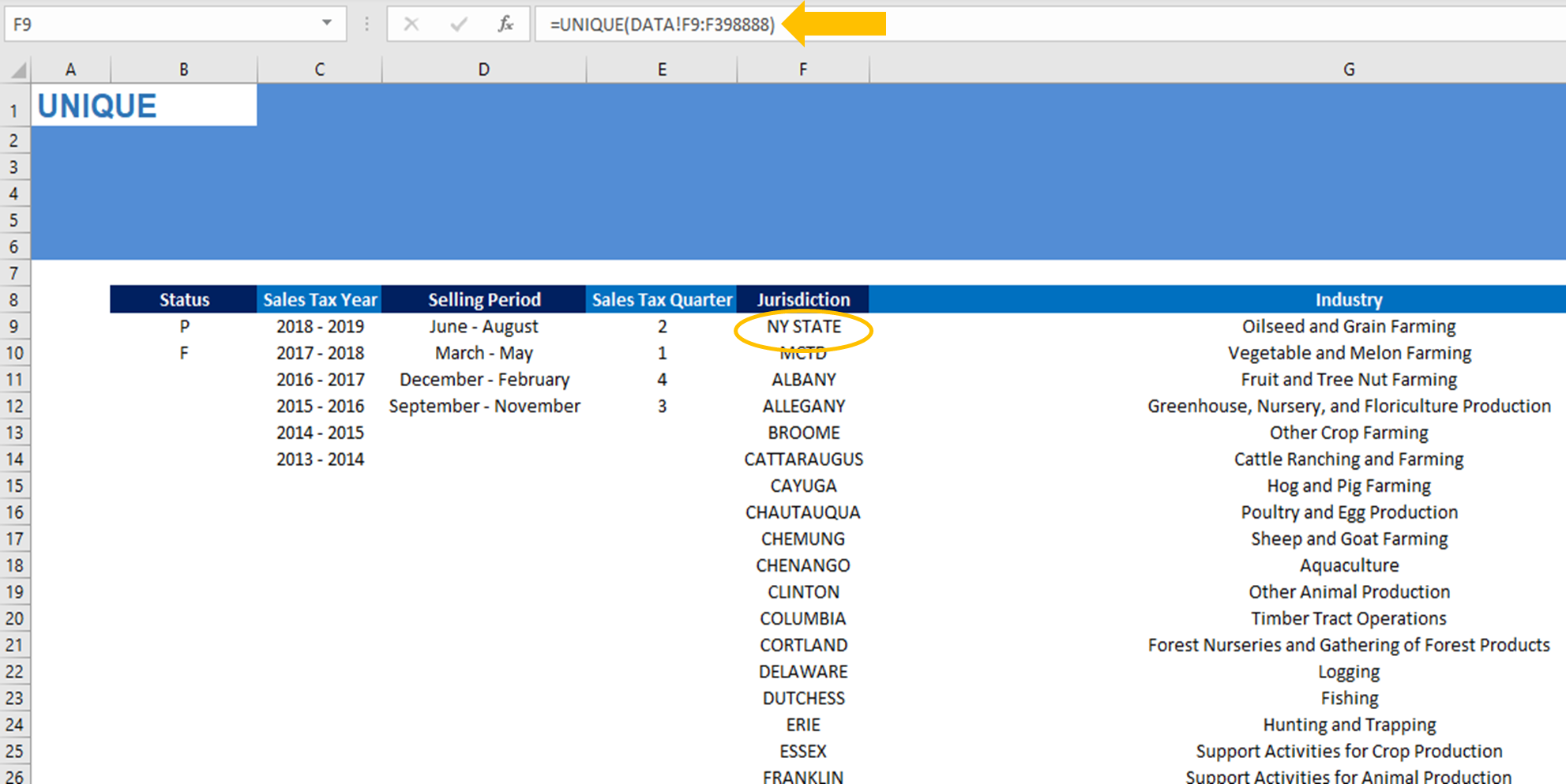When data changes the spill range (the area covered with data) will expand or contract as needed.

You might see new values added or existing values removed (in Table 2) based on the changes in Table 1.

HASH SYMBOL

To refer to a spill range you can use the hash symbol (#). For example, if we wanted to know the number of unique jurisdictions, we could use the following formula to get the count of unique jurisdictions. The result is updated based on the spill range of the unique formula. This makes the COUNT formula dynamic, without the need for the user to update the formula range.SEQUENCE

Another useful function is SEQUENCE. See the example below where we need a series of numbers over 12 columns and 1 row, where we start from 1 and increase each time by 1 (i.e. the twelve months of the year). We use only 1 formula to produce range C13:N13.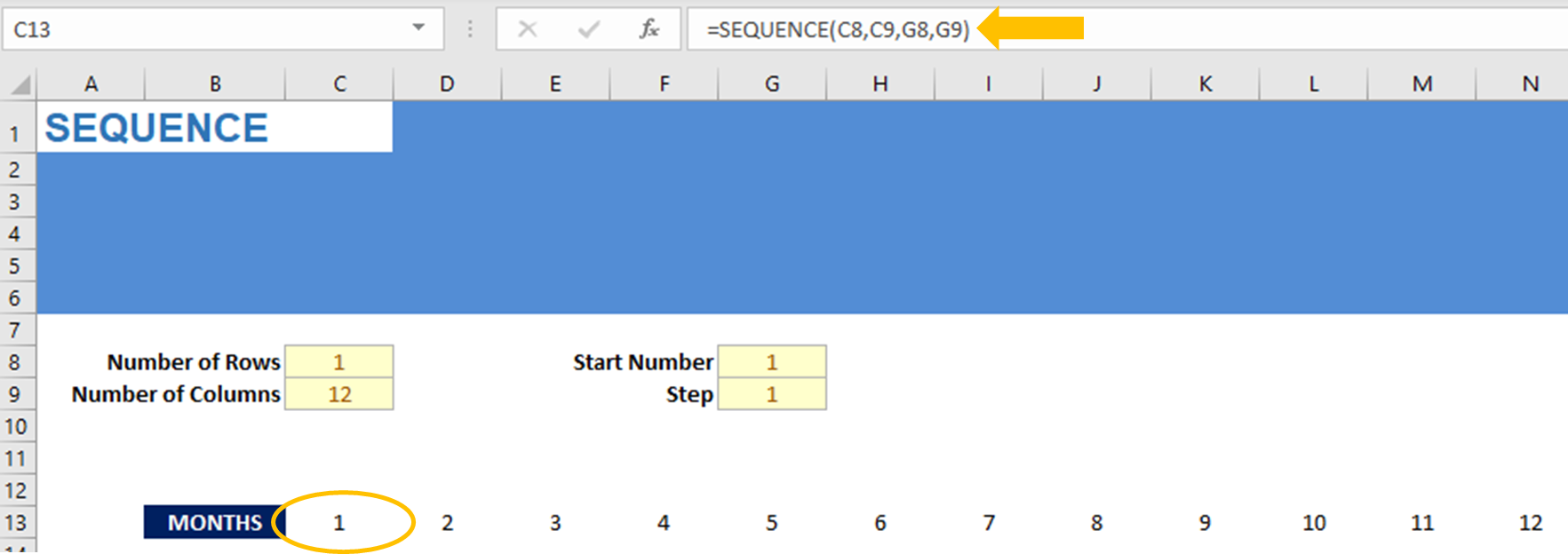SORT

Returning to our Table 2 example with jurisdictions, how could we sort this range without applying filters? We can use the SORT function as per the example below and combine it with the hash symbol (#):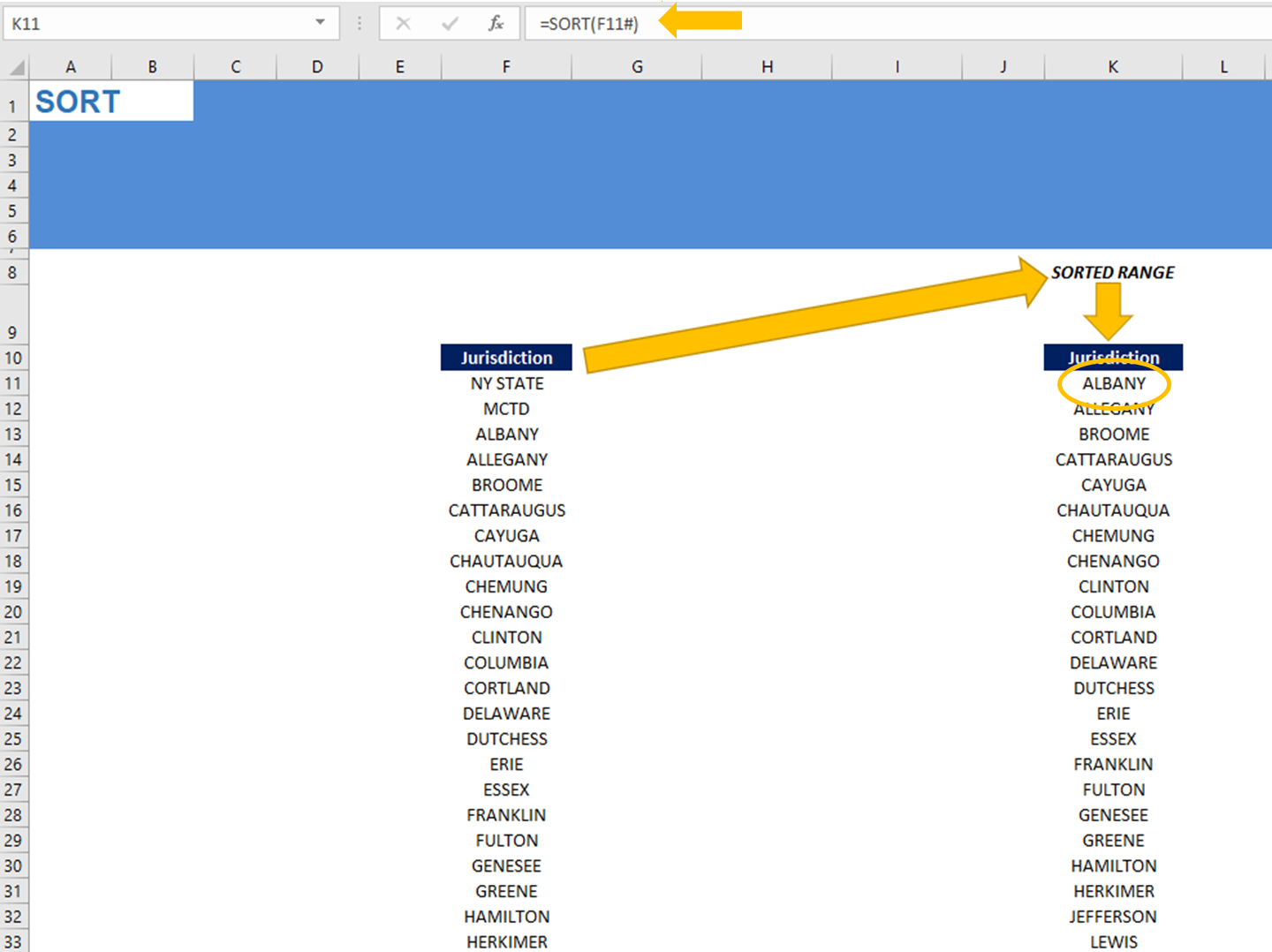SORTBY

Let us assume, we would like to sort based on multiple criteria. For example, by fiscal year, then by jurisdiction, and then by industry. We would then have to use the SORTBY function, which would sort our initial data in Table 1 with those criteria. Again only 1 formula is used for this.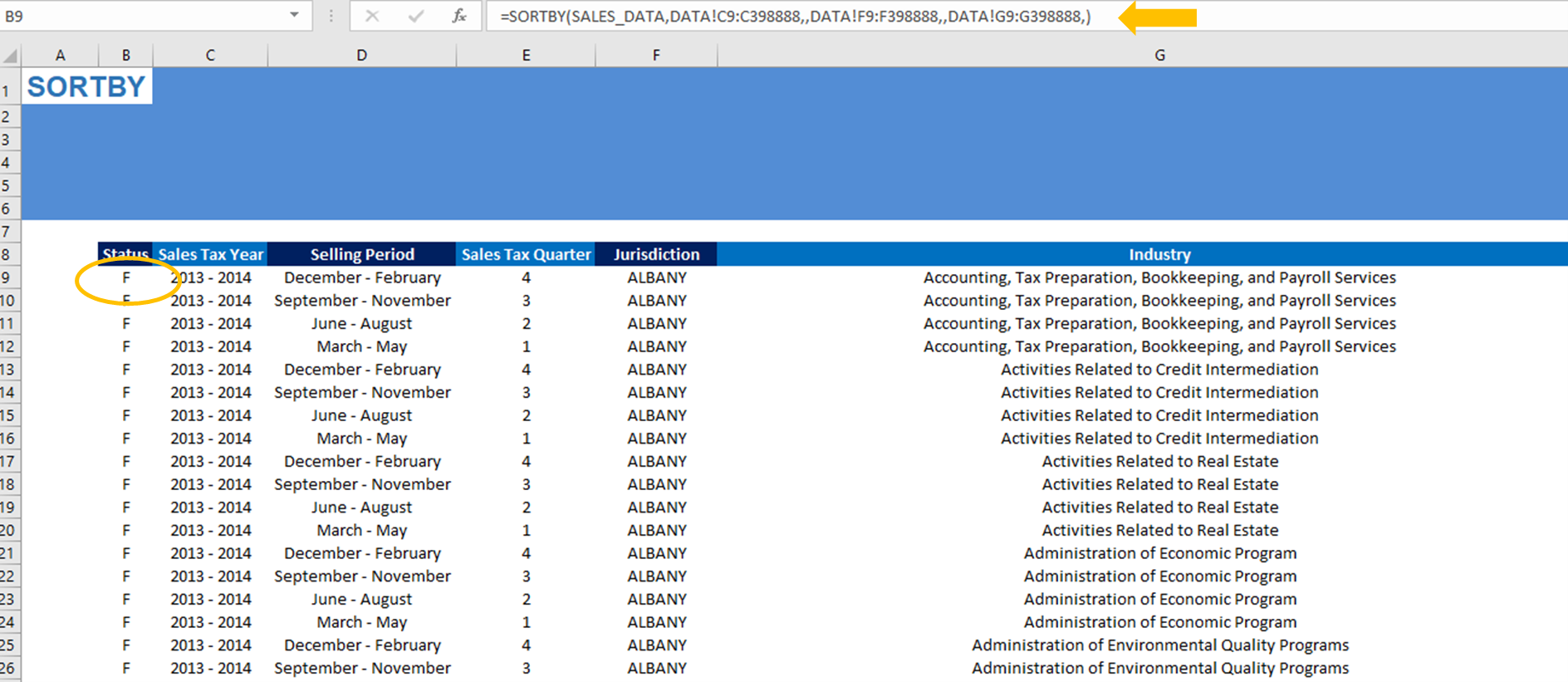RANDARRAY

There are cases where we need an array of random numbers (for simulation purposes for example).

We can produce such a random array of numbers based on the formula RANDARRAY. See the example below where we produce a 10 by 10 array with random numbers from 1 to 10, with only one formula.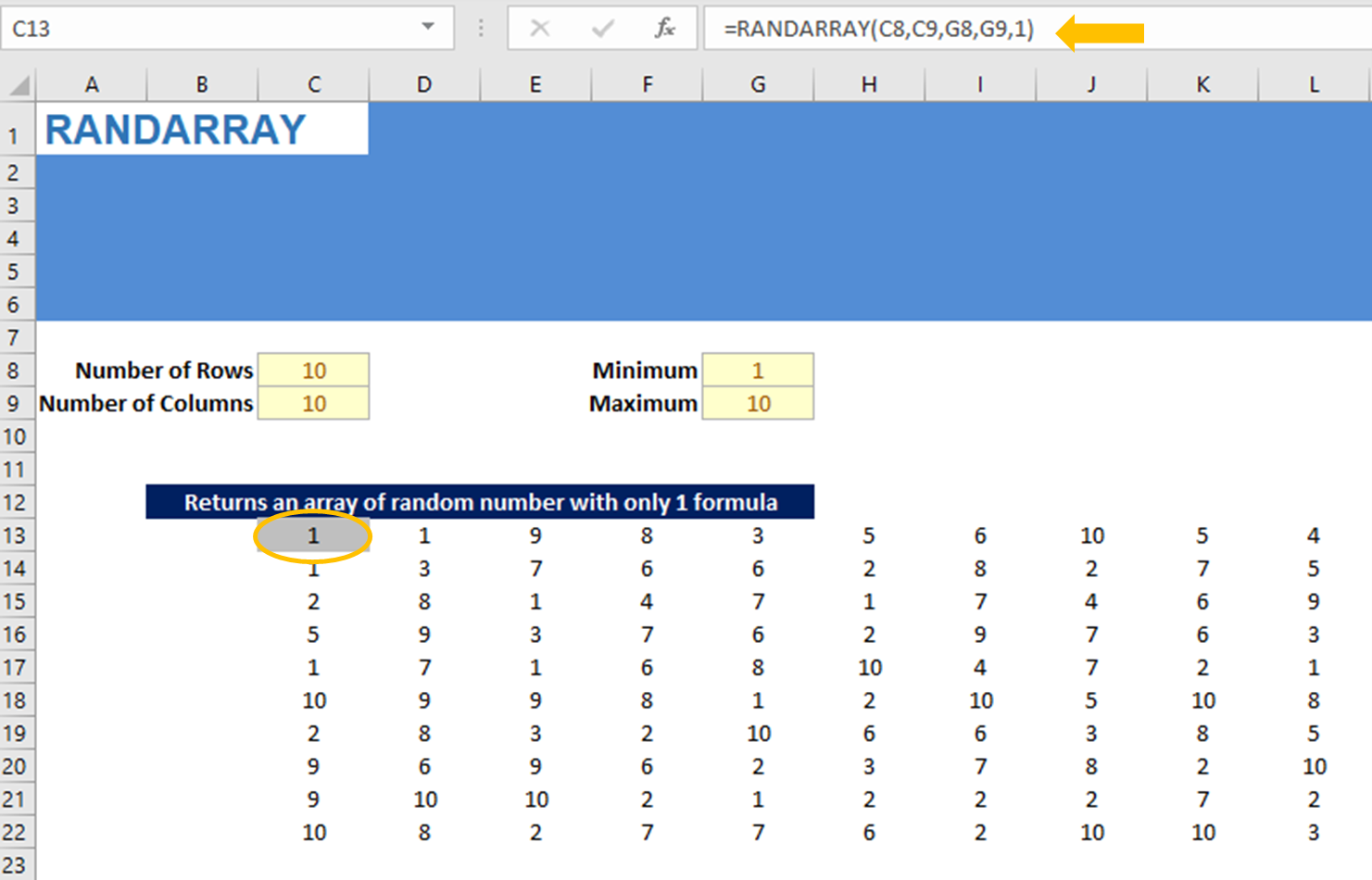FILTER

One of the most interesting formulas to work with arrays is the filter formula. This formula allows the user to create a pivot table, but with a formula instead of using the ribbon menu.LAST BUT NOT LEAST

Finally we can also use arrays with simple operators such as additions, subtractions, multiplications and divisions (+ ,  - , *  , / ). See the example below for multiplications and subtractions.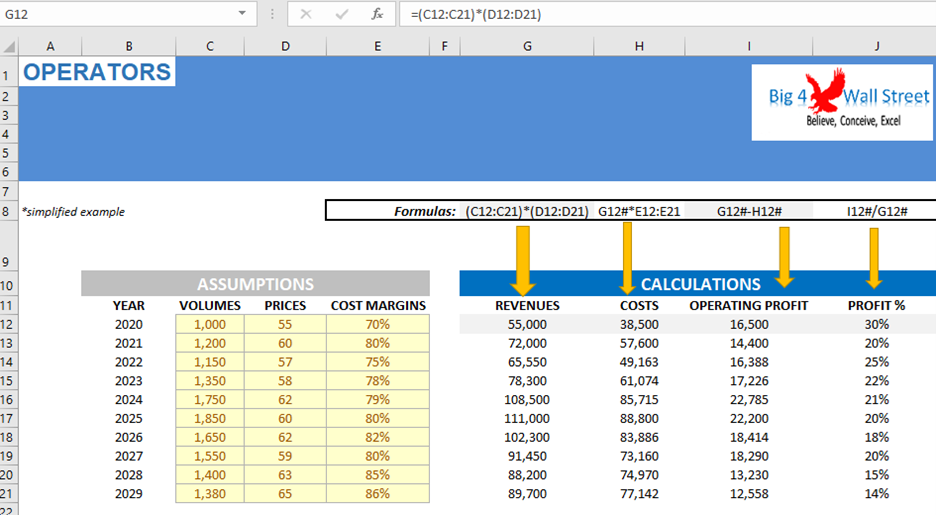In the above example, only 4 formulas are used. One formula in cell G12 for the Revenues, one in H12 for Costs, one in I12 for Operating Profit and one in J12 for Profit Margin.

Comparing this with “traditional” excel formulas, we would have to use 40 formulas to produce the same results. Even if we increased the years to 40 years, we would still use 4 formulas with dynamic ranges, instead of 160 formulas with traditional excel formulas.

This makes models easier to audit, saves time, and avoids errors.

Check the free file with the worked examples here.